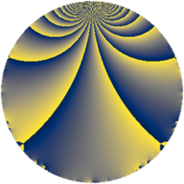# Properties

 Label 230.2.bLevel $230$ Weight $2$ Character orbit 230.b Rep. character $\chi_{230}(139,\cdot)$ Character field $\Q$ Dimension $12$ Newform subspaces $2$ Sturm bound $72$ Trace bound $1$

# Related objects

## Defining parameters

 Level: $$N$$ $$=$$ $$230 = 2 \cdot 5 \cdot 23$$ Weight: $$k$$ $$=$$ $$2$$ Character orbit: $$[\chi]$$ $$=$$ 230.b (of order $$2$$ and degree $$1$$) Character conductor: $$\operatorname{cond}(\chi)$$ $$=$$ $$5$$ Character field: $$\Q$$ Newform subspaces: $$2$$ Sturm bound: $$72$$ Trace bound: $$1$$ Distinguishing $$T_p$$: $$3$$

## Dimensions

The following table gives the dimensions of various subspaces of $$M_{2}(230, [\chi])$$.

Total New Old
Modular forms 40 12 28
Cusp forms 32 12 20
Eisenstein series 8 0 8

## Trace form

 $$12q - 12q^{4} + 4q^{6} - 8q^{9} + O(q^{10})$$ $$12q - 12q^{4} + 4q^{6} - 8q^{9} - 8q^{11} - 16q^{15} + 12q^{16} + 16q^{19} - 4q^{24} + 8q^{25} + 4q^{26} + 8q^{29} - 12q^{31} - 16q^{35} + 8q^{36} - 8q^{39} + 28q^{41} + 8q^{44} - 16q^{45} + 4q^{46} - 8q^{49} - 12q^{50} + 24q^{51} - 16q^{54} - 8q^{55} - 8q^{59} + 16q^{60} + 16q^{61} - 12q^{64} - 16q^{65} + 8q^{66} - 8q^{69} + 20q^{70} + 44q^{71} - 32q^{75} - 16q^{76} + 16q^{79} + 28q^{81} - 24q^{85} - 40q^{86} - 48q^{89} - 24q^{90} - 32q^{91} - 24q^{94} + 48q^{95} + 4q^{96} + 32q^{99} + O(q^{100})$$

## Decomposition of $$S_{2}^{\mathrm{new}}(230, [\chi])$$ into newform subspaces

Label Dim. $$A$$ Field CM Traces $q$-expansion
$$a_2$$ $$a_3$$ $$a_5$$ $$a_7$$
230.2.b.a $$4$$ $$1.837$$ $$\Q(i, \sqrt{5})$$ None $$0$$ $$0$$ $$0$$ $$0$$ $$q-\beta _{3}q^{2}+\beta _{1}q^{3}-q^{4}+(1+2\beta _{2})q^{5}+\cdots$$
230.2.b.b $$8$$ $$1.837$$ 8.0.$$\cdots$$.3 None $$0$$ $$0$$ $$0$$ $$0$$ $$q-\beta _{2}q^{2}+(\beta _{2}-\beta _{5})q^{3}-q^{4}+(\beta _{1}-\beta _{6}+\cdots)q^{5}+\cdots$$

## Decomposition of $$S_{2}^{\mathrm{old}}(230, [\chi])$$ into lower level spaces

$$S_{2}^{\mathrm{old}}(230, [\chi]) \cong$$ $$S_{2}^{\mathrm{new}}(115, [\chi])$$$$^{\oplus 2}$$